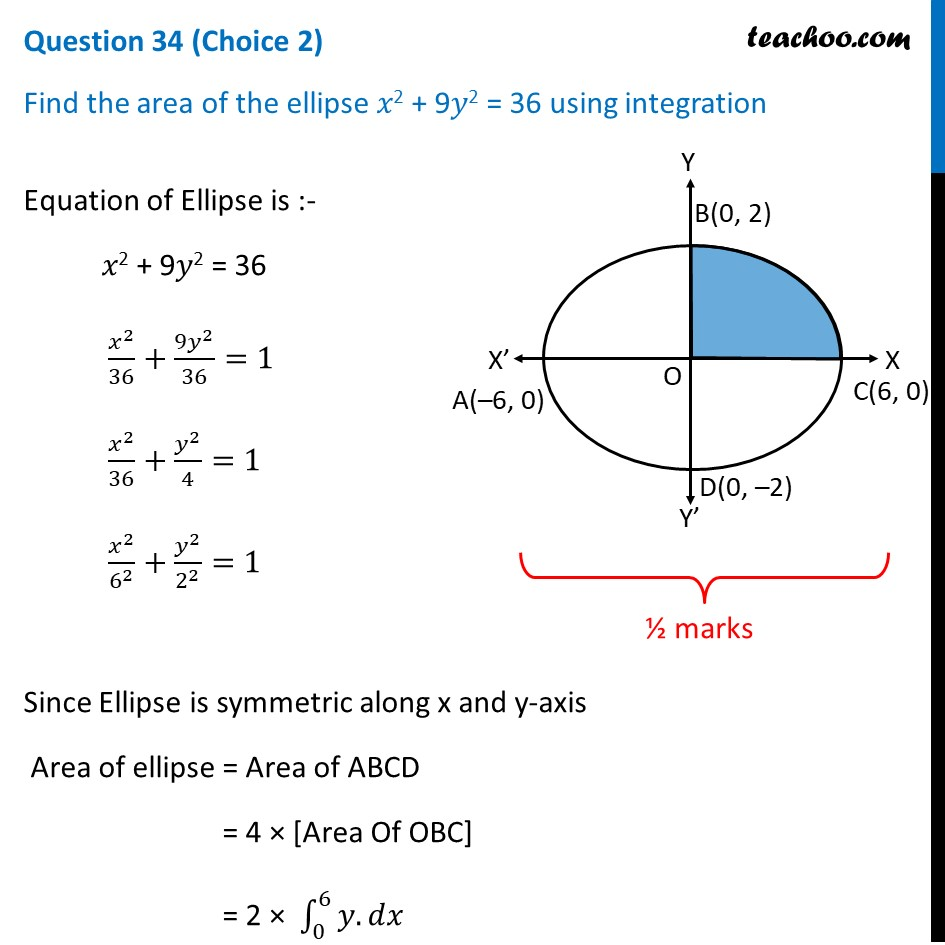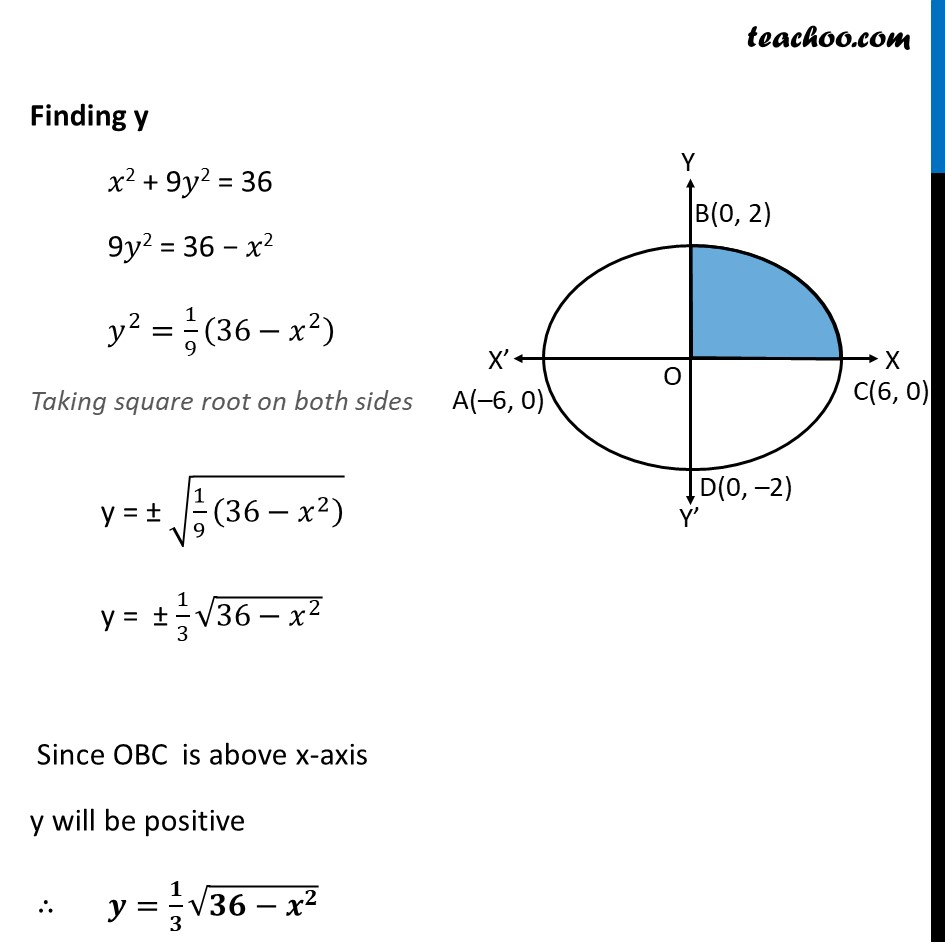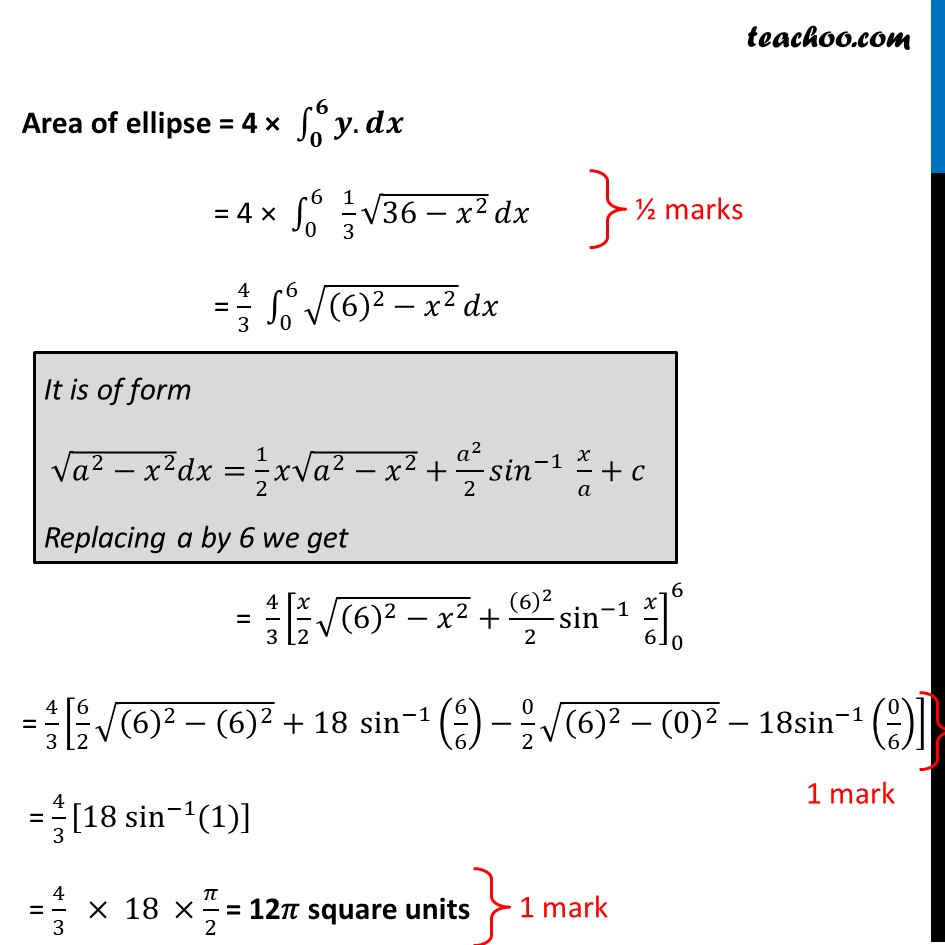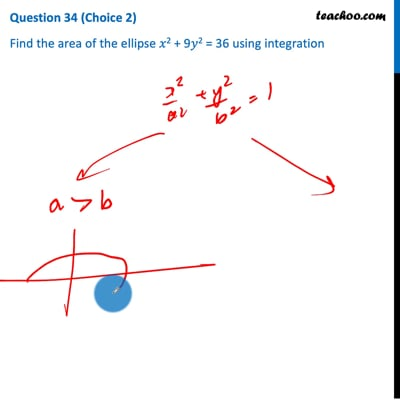CBSE Class 12 Sample Paper for 2021 Boards

Class 12
Solutions of Sample Papers and Past Year Papers - for Class 12 Boards

## Find the area of the ellipse 𝑥 2 + 9𝑦 2 = 36 using integrationNote : This is similar to Ex 8.1, 4 of NCERT – Chapter 8 Class 12 Application of Integration

Check the answer here https:// www.teachoo.com /3328/730/Ex-8.1--4---Find-area-bounded-by-ellipse-x2-16---y2-9--1/category/Ex-8.1/This video is only available for Teachoo black users

Introducing your new favourite teacher - Teachoo Black, at only ₹83 per month

### Transcript

Question 34 (Choice 2) Find the area of the ellipse 𝑥2 + 9𝑦2 = 36 using integration Equation of Ellipse is :- 𝑥2 + 9𝑦2 = 36 𝑥^2/36+(9𝑦^2)/36=1 𝑥^2/36+𝑦^2/4=1 𝑥^2/6^2 +𝑦^2/2^2 =1 Since Ellipse is symmetric along x and y-axis Area of ellipse = Area of ABCD = 4 × [Area Of OBC] = 2 × ∫_0^6▒〖𝑦.〗 𝑑𝑥 Finding y 𝑥2 + 9𝑦2 = 36 9𝑦2 = 36 − 𝑥2 𝑦^2=1/9 (36−𝑥^2 ) Taking square root on both sides y = ± √(1/9 (36−𝑥^2 ) ) y = ± 1/3 √(36−𝑥^2 ) Since OBC is above x-axis y will be positive ∴ 𝒚=𝟏/𝟑 √(𝟑𝟔−𝒙^𝟐 ) Area of ellipse = 4 × ∫_𝟎^𝟔▒〖𝒚.〗 𝒅𝒙 = 4 × ∫_0^6▒〖 1/3 √(36−𝑥^2 )〗 𝑑𝑥 = 4/3 ∫_0^6▒√((6)^2−𝑥^2 ) 𝑑𝑥 It is of form √(𝑎^2−𝑥^2 ) 𝑑𝑥=1/2 𝑥√(𝑎^2−𝑥^2 )+𝑎^2/2 〖𝑠𝑖𝑛〗^(−1)⁡〖 𝑥/𝑎+𝑐〗 Replacing a by 6 we get = 4/3 [𝑥/2 √((6)^2−𝑥^2 )+(6)^2/2 sin^(−1)⁡〖 𝑥/6〗 ]_0^6 = 4/3 [6/2 √((6)^2−(6)^2 )+18 〖 sin〗^(−1)⁡(6/6)−0/2 √((6)^2−(0)^2 )−18sin^(−1) (0/6)] = 4/3 [18 sin^(−1) (1)] = 4/3 × 18 ×𝜋/2 = 12𝜋 square units